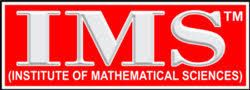# Live Online/Offline Classes by : K. Venkanna

##### (For Paper I & Paper II)
 Book Name Download Link 1. Linear Algebra ● Linear Algebra by A.R.Vasista● Linear Algebra by  Schaum Series (3000 solved problems book) 2. Calculus and Real Analysis ● Calculus and Real Analysis by Shanti Narayana 3. 3-D Geometry ● 3-D Geometry by P.N.Chatterjee 4. Ordinary Differential Equations ● Vector Analysis by A.R. Vasista● Vector Analysis by Schuam Series 5. Vector Analysis ● Vector Analysis by A.R. Vasista● Vector Analysis by Schuam Series 6. Algebra ● Algebra by Joseph A. Gallian● Algebra by Shramik Sen Upadhayay 7. Complex Analysis ● Complex Analysis by Schuam Series● Complex Analysis by J.N. Sharma● Complex Analysis by Ponnu Swami 8. Linear Programming ● Linear Programming by Shanti Swarup● Linear Programming by Kanti Swarup 9. Numerical Analysis ● Numerical Analysis by Jain and Iyenger● Numerical Analysis by K. Shankar Rao● Numerical Analysis by S.S. Shasthry 10. Computer Programming ● Computer Programming by Raja Raman 11. Dynamics & Statics ● Dynamics & Statics by A.R. Vasista● Dynamics & Statics by M. Ray 12. Mechanics and Fluid Dynamics ● Mechanics and Fluid Dynamics by M.D. Raisinghania● Mechanics and Fluid Dynamics by R.K. Gupta● Mechanics and Fluid Dynamics by J.K. Goyal and K.P. Gupta